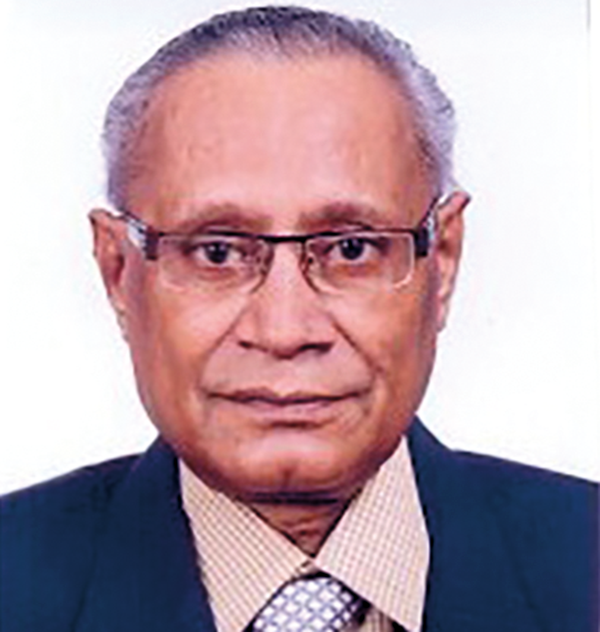19

June , 2017
Aryabhatta, the master mathematician-astronomer-sage
16:34 pm

B.E. BureauArybhatta was an ancient mathematician and astronomer of India. He was born in Pataliputra (Magadh) in Bihar. Some opine that he may have been born in Kerala. Arya is a south Indian name whereas the name Bhatta has a north Indian origin. Whatever be the controversies, it is speculated that he was the head of the Nalanda University and established the astronomical observatory there and composed his famous book in Sanskrit known as the “Aryabhatiya” at the age of 23.

This book has 108 verses with 13 introductory verses comprised of 4 chapters:

1)   Gitikapada ― containing large period of times Kalpa, Manvantra, Yuga and Mahayuga where Mahayuga is 4.32 million years.

2)   Ganitapada ― includes A.P.; G.P.; equations, diophantine (indeterminate) equations etc.

3)   Kalakriyapada ― Defined the solar system and deduced the Hindu Calendar.

4)   Golapada- Geometric and trigonometrical aspects of celestial spheres etc. Amongst his many important works, some of the most outstanding were

a)   Value of Pi (π) = circumference/diameter in a circle:
He deduced, [{(4+100) x 8}+62000]÷ 20,000=3.1416 (approx) where the diameter of a circle is 20,000.

This brilliant finding of incommensurable value of π was proved by Lambart in 1761.

b)  Place value system and outstanding idea of zero:

For the first time, he gave the idea of zero, which was unknown till then. It was later on acknowledged by the French mathematician Georges Ifrah that Aryabhatta was the first mathematician to give the concept of zero. He also stated the initial 10 decimal places. He also gave the methods of finding square roots, cube roots and summations of (1+2+3+……), (12+22+32+……), (13+23+33+……),(14 +24+34+……), etc. The formula of (a+b)2 was also devised by him.

c)    Computation of the earth’s circumference:

He first computed it as 24835 miles as compared to modern value of 24900 miles. He also stated that the earth was spherical and rotating about its axis causing day and night. He worked out a year to be 365 days and 7 days a week with the idea of a leap year. He found out the positions of nine planets revolving around the sun and the causes of eclipses. He also discovered that these planets do not emit their own light. He also opined that the universe has many celestial systems by establishing Astro – geometric and Astro-trigonometric formulae and established the idea of spherical angles, which is still being followed in recent times.

d)    Indeterminate equations:

His method of solving diophantine equations by using the Kuttaka method (breaking into small pieces) was exceptional.

Bhaskara’s thought process – find the number which gives 5 as reminder when divided by 8; 4 as reminder when divided by 9 and 1 as reminder when divided by 7 i.e, find P=8x+5=9y+4=7z+1 which turns out to smallest value of P as 85.

e)    In Ganitapada 6, he gave the area of right angled triangle as “a  perpendicular with half the side”.

f)    He defined the concept of sine as “ardha-jya” –half chord.

Aryabhatta also felt that all particles cling to the surface of the earth for some unknown reason but did not mention the attractive force. Later on, his follower Brahmagupta was more specific regarding this attraction. Newton, while discovering it opined that he did it “by standing on the shoulders of the giants”. Aryabhatta and Brahmagupta may be those giants.

Aryabhatta’s works were later adopted by Greeks and Arabs. His book, Aryabhatiya was translated into Latin in the 13th century and European mathematicians learnt various methods from it.

—  The author is an Emeritus Professor & Chairman of IQAC
and member secretary of the Hooghly Engineering and Technology College